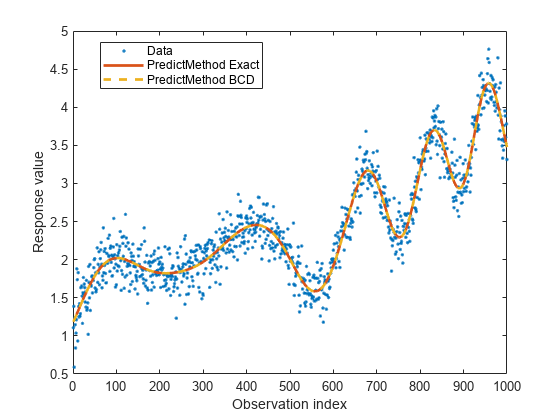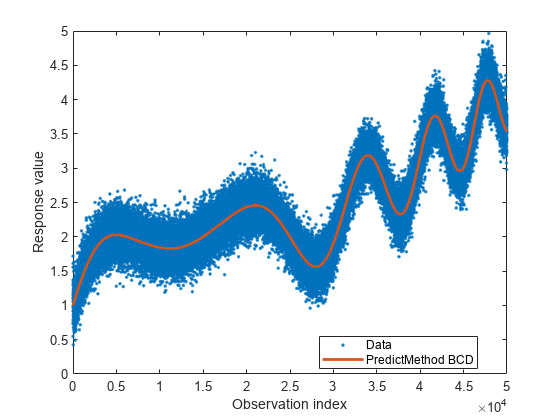## Block Coordinate Descent Approximation for GPR Models

For a large number of observations, using the exact method for parameter estimation and making predictions on new data can be expensive (see Exact GPR Method). One of the approximation methods that help deal with this issue for prediction is the Block Coordinate Descent (BCD) method. You can make predictions using the BCD method by first specifying the predict method using the 'PredictMethod','bcd' name-value pair argument in the call to fitrgp, and then using the predict function.

The idea of the BCD method is to compute

$\stackrel{^}{\alpha }={\left(K\left(X,X\right)+{\sigma }^{2}{I}_{N}\right)}^{-1}\left(y-H\beta \right)$

in a different way than the exact method. BCD estimates $\stackrel{^}{\alpha }$by solving the following optimization problem:

where

$f\left(\alpha \right)=\frac{1}{2}{\alpha }^{T}\left[K\left(X,X\right)+{\sigma }^{2}{I}_{N}\right]\alpha -{\alpha }^{T}\left(y-H\beta \right).$

fitrgp uses block coordinate descent (BCD) to solve the above optimization problem. For users familiar with support vector machines (SVMs), sequential minimal optimization (SMO) and ISDA (iterative single data algorithm) used to fit SVMs are special cases of BCD. fitrgp performs two steps for each BCD update - block selection and block update. In the block selection phase, fitrgp uses a combination of random and greedy strategies for selecting the set of $\alpha$ coefficients to optimize next. In the block update phase, the selected $\alpha$ coefficients are optimized while keeping the other $\alpha$ coefficients fixed to their previous values. These two steps are repeated until convergence. BCD does not store the $n*n$ matrix $K\left(X,X\right)$ in memory but it just computes chunks of the $K\left(X,X\right)$ matrix as needed. As a result BCD is more memory efficient compared to 'PredictMethod','exact'.

Practical experience indicates that it is not necessary to solve the optimization problem for computing $\alpha$ to very high precision. For example, it is reasonable to stop the iterations when $||\nabla f\left(\alpha \right)||$ drops below $\eta ||\nabla f\left({\alpha }_{0}\right)||$, where ${\alpha }_{0}$ is the initial value of $\alpha$ and $\eta$ is a small constant (say $\eta ={10}^{-3}$). The results from 'PredictMethod','exact' and 'PredictMethod','bcd' are equivalent except BCD computes $\alpha$ in a different way. Hence, 'PredictMethod','bcd' can be thought of as a memory efficient way of doing 'PredictMethod','exact'. BCD is often faster than 'PredictMethod','exact' for large n. However, you cannot compute the prediction intervals using the 'PredictMethod','bcd' option. This is because computing prediction intervals requires solving a problem like the one solved for computing $\alpha$ for every test point, which again is expensive.

### Fit GPR Models Using BCD Approximation

This example shows fitting a Gaussian Process Regression (GPR) model to data with a large number of observations, using the Block Coordinate Descent (BCD) Approximation.

Small n - PredictMethod 'exact' and 'bcd' Produce the Same Results

Generate a small sample dataset.

 rng(0,'twister'); n = 1000; X = linspace(0,1,n)'; X = [X,X.^2]; y = 1 + X*[1;2] + sin(20*X*[1;-2])./(X(:,1)+1) + 0.2*randn(n,1); 

Create a GPR model using 'FitMethod','exact' and 'PredictMethod','exact'.

 gpr = fitrgp(X,y,'KernelFunction','squaredexponential',... 'FitMethod','exact','PredictMethod','exact'); 

Create another GPR model using 'FitMethod','exact' and 'PredictMethod','bcd'.

 gprbcd = fitrgp(X,y,'KernelFunction','squaredexponential',... 'FitMethod','exact','PredictMethod','bcd','BlockSize',200); 

'PredictMethod','exact' and 'PredictMethod','bcd' should be equivalent. They compute the samebut just in different ways. You can also see the iterations by using the 'Verbose',1 name-value pair argument in the call to fitrgp .

Compute the fitted values using the two methods and compare them:

 ypred = resubPredict(gpr); ypredbcd = resubPredict(gprbcd); max(abs(ypred-ypredbcd)) 
ans = 5.8853e-04 

The fitted values are close to each other.

Now, plot the data along with fitted values for 'PredictMethod','exact' and 'PredictMethod','bcd'.

 figure; plot(y,'k.'); hold on; plot(ypred,'b-','LineWidth',2); plot(ypredbcd,'m--','LineWidth',2); legend('Data','PredictMethod Exact','PredictMethod BCD','Location','Best'); xlabel('Observation index'); ylabel('Response value');It can be seen that 'PredictMethod','exact' and 'PredictMethod','bcd' produce nearly identical fits.

Large n - Use 'FitMethod','sd' and 'PredictMethod','bcd'

Generate a bigger dataset similar to the previous one.

 rng(0,'twister'); n = 50000; X = linspace(0,1,n)'; X = [X,X.^2]; y = 1 + X*[1;2] + sin(20*X*[1;-2])./(X(:,1)+1) + 0.2*randn(n,1); 

For n = 50000, the matrix K(X, X) would be 50000-by-50000. Storing K(X, X) in memory would require around 20 GB of RAM. To fit a GPR model to this dataset, use 'FitMethod','sd' with a random subset of m = 2000 points. The 'ActiveSetSize' name-value pair argument in the call to fitrgp specifies the active set size m. For computinguse 'PredictMethod','bcd' with a 'BlockSize' of 5000. The 'BlockSize' name-value pair argument in fitrgp specifies the number of elements of thevector that the software optimizes at each iteration. A 'BlockSize' of 5000 assumes that the computer you use can store a 5000-by-5000 matrix in memory.

 gprbcd = fitrgp(X,y,'KernelFunction','squaredexponential',..., 'FitMethod','sd','ActiveSetSize',2000,'PredictMethod','bcd','BlockSize',5000); 

Plot the data and the fitted values.

 figure; plot(y,'k.'); hold on; plot(resubPredict(gprbcd),'m-','LineWidth',2); legend('Data','PredictMethod BCD','Location','Best'); xlabel('Observation index'); ylabel('Response value');The plot is similar to the one for smaller n .

 Grippo, L. and M. Sciandrone. "On the convergence of the block nonlinear gauss-seifel method under convex constraints." Operations Research Letters. Vol. 26, pp. 127–136, 2000.

 Bo, L. and C. Sminchisescu. "Greed block coordinate descent for large scale Gaussian process regression." In Proceedings of the Twenty Fourth Conference on Uncertainty in Artificial Intelligence (UAI2008): http://arxiv.org/abs/1206.3238, 2012.